Learning Objective

In this lesson we will learn how ionic formulas reflect the ratio of positive and negative ions in ionic compounds.

Learning Outcomes

By the end of this lesson you will be able to:

• Write the formula for ionic compounds containing only monatomic ions.

• Write the formula for ionic compounds containing polyatomic ions.

BUY THE YEAR 9 CHEMISTRY WORKBOOK

## Ionic Compounds

• Like elements, compounds have no overall charge.
This is because they have equal numbers of protons and electrons.
• Ionic compounds are a type of compound composed of charged particles called ions.
For ionic compounds to be neutral, the total amount of positive ion charge must equal the total amount of negative ion charge.

## Ionic Formulas

• Ionic compounds are not composed of discrete molecules, but are instead made up of continuous lattices of positive and negative ions.
The formulas for ionic compounds therefore do not represent molecules, but instead represent the ratio of the positive and negative ions in the compound.
• Example
The formula for magnesium chloride is MgCl2.
This shows that the compound is made up of magnesium ions and chloride ions in a 1:2 ratio.
This is because, since magnesium ions have a +2 charge and chloride ions have a –1 charge, there needs to be twice as many chloride ions as magnesium ions for there to be no overall charge.
(Remember, that if there is no subscript number to the bottom right of an element’s symbol in a compound’s formula, there is one copy of that atom in the molecule or ionic ratio.)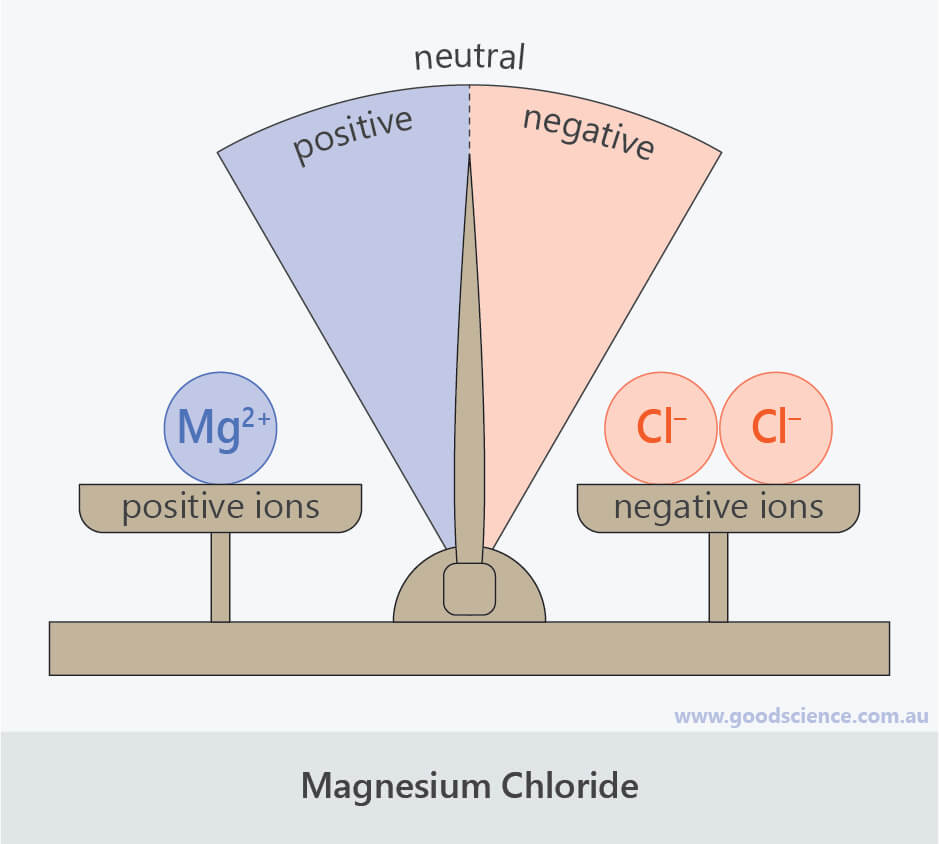In ionic compounds, the total amount of positive ion charge equals the total amount of negative ion charge.

## The ‘Drag-and-Drop’ Method for Determining Ionic Formulas

• The ‘drag-and-drop’ method is a shorthand way of determining the formula for ionic compounds.
By following a series of steps, the formula for any ionic compound can be determined.
It is best illustrated using examples.

### Ionic Compounds Containing only Monatomic Ions

Example

Determining the ionic formula for aluminium oxide.

Step 1

Determine the charges and symbols of the ions making up the compound.

For ions of main group elements, the charge can be determined by what group the element is in on the periodic table (see the lesson “Formation of Ions and Ionic Compounds”).

Aluminium is in group 13, therefore aluminium ions have a charge of +3 and the symbol Al3+.

Oxygen is in group 16, therefore oxygen ions have a charge of –2 and the symbol O2–.

Step 2

Write the positive and negative ions next to each other, with a space in between.Step 3

‘Drag’ the number in front of each charge diagonally downwards and ‘drop’ it on the bottom right side (subscript) of the other ion.

Drag the number only, leave the sign. Remember that 1s are ‘invisible’ and therefore stay that way.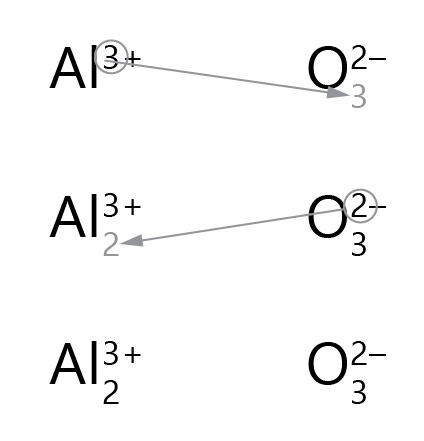Step 4

Remove the original number and sign of the charge on each ion.Step 5

Join the two parts together.

This is the formula of the ionic compound.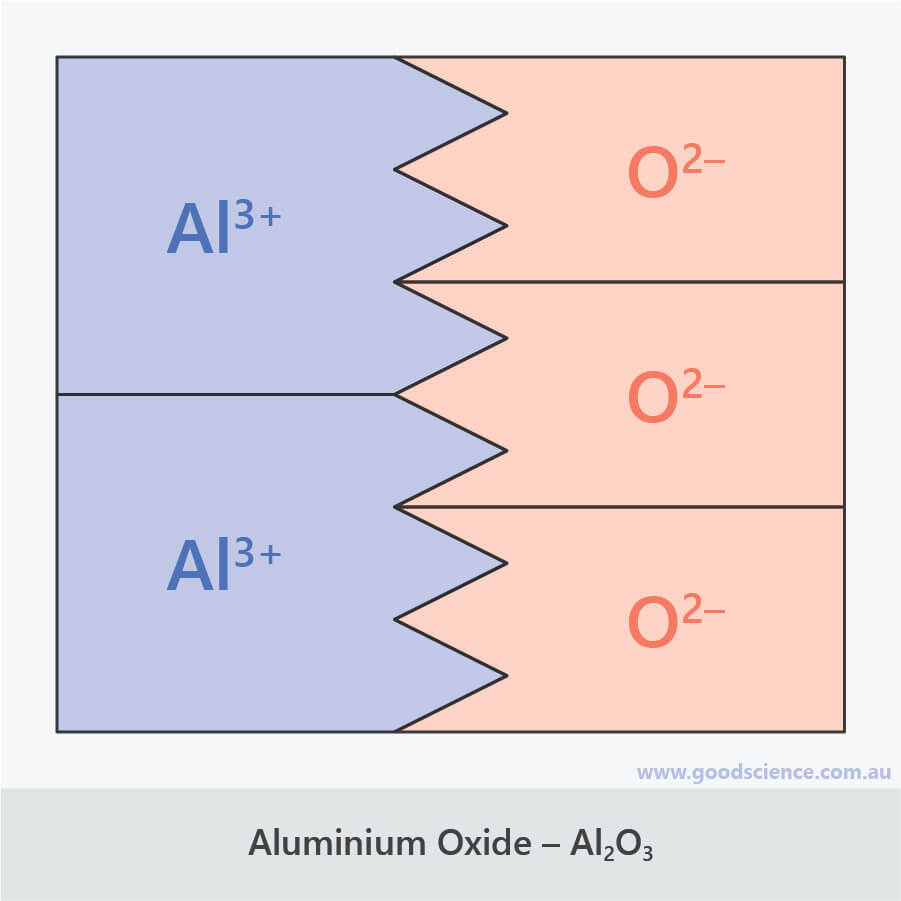The formula for aluminium oxide shows that the compound is composed of aluminium ions and oxide ions in a 2:3 ratio.

### Ionic Compounds Containing Polyatomic Ions

Example

Determining the ionic formula for iron (II) phosphate.

Step 1

Determine the charges and symbols of the ions making up the compound.

For transition metal ions, the charge can be determined from the Roman numeral after the name (see the lesson “Formation of Ions and Ionic Compounds”).

Iron (II) has a charge of +2 and the symbol Fe2+.

For polyatomic ions, the charge and symbol can be determined by consulting a valency table.

Phosphate has a charge of –3 and the symbol PO43–.

Step 2

Write the positive and negative ions next to each other, with a space in between.Step 3

‘Drag-and-drop’ as above for monatomic ions.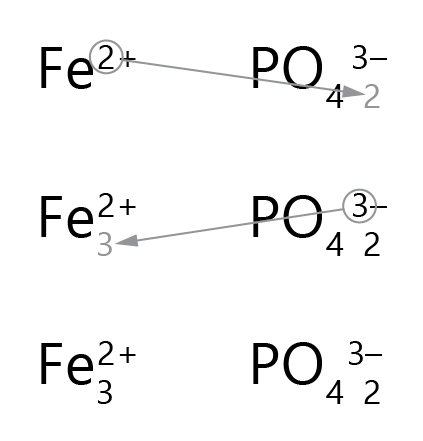Step 4

Remove the original number and sign of the charge on each ion.Step 5

Place brackets around any polyatomic ions that now have a dragged number (subscript) next to them.

The dragged number should be outside the brackets.Step 6

Join the two parts together.

This is the formula of the ionic compound.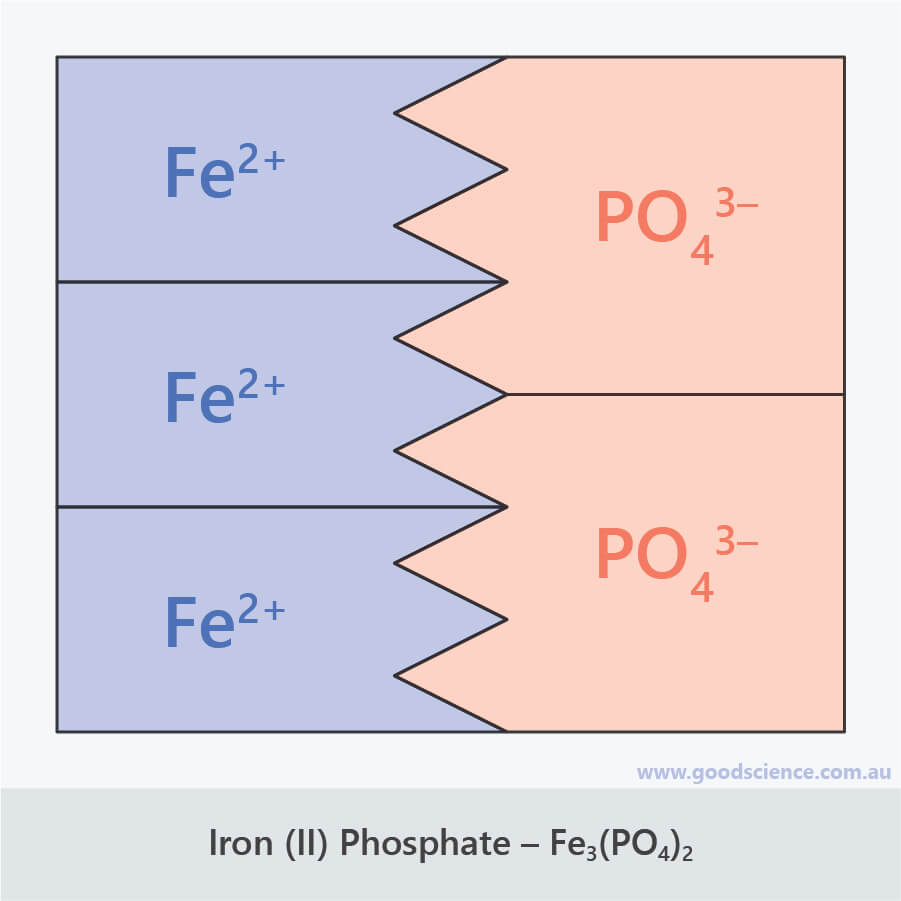The formula for iron (II) phosphate shows that the compound is composed of iron (II) ions and phosphate ions in a 3:2 ratio.

### Exceptions to the Drag-and-Drop Rule

• There is one exception to the drag-and-drop rule:
Whenever the size of the charge is the same for each ion, simply write the ionic formula of the compound as a 1:1 ratio.
This is because ionic formulas are always written as the simplest ratio of positive and negative ions.
Example 1
For the ions Mg2+ and O2–, the formula of the compound formed is MgO, not Mg2O2.
Example 2
For the ions Al3+ and PO43–, the formula of the compound formed is AlPO4, not Al3(PO4)3.

## Summary

• Although ionic compounds are composed of charged particles (ions), they have no overall charge.
This is because the total amount of positive charge equals the total amount of negative charge.
• The formulas for ionic compounds do not represent molecules – they represent the ratio of positive and negative ions in the compound’s lattice structure.
• The ‘drag-and-drop’ method is a shorthand way of determining the formula for ionic compounds.
It involves ‘dragging’ the number in front of each ion and ‘dropping’ it on the bottom right side (subscript) of the other ion.
• When there is more than one copy of a polyatomic ion in a formula, it needs to have brackets around it.
• When the size of the charge is the same for each ion in a compound (+1 and –1, +2 and –2, or +3 and –3), the drag-and-drop rule does not apply.
The formula of the compound is simply a 1:1 ratio of the two ions.(Image: Vacho, Pixabay)